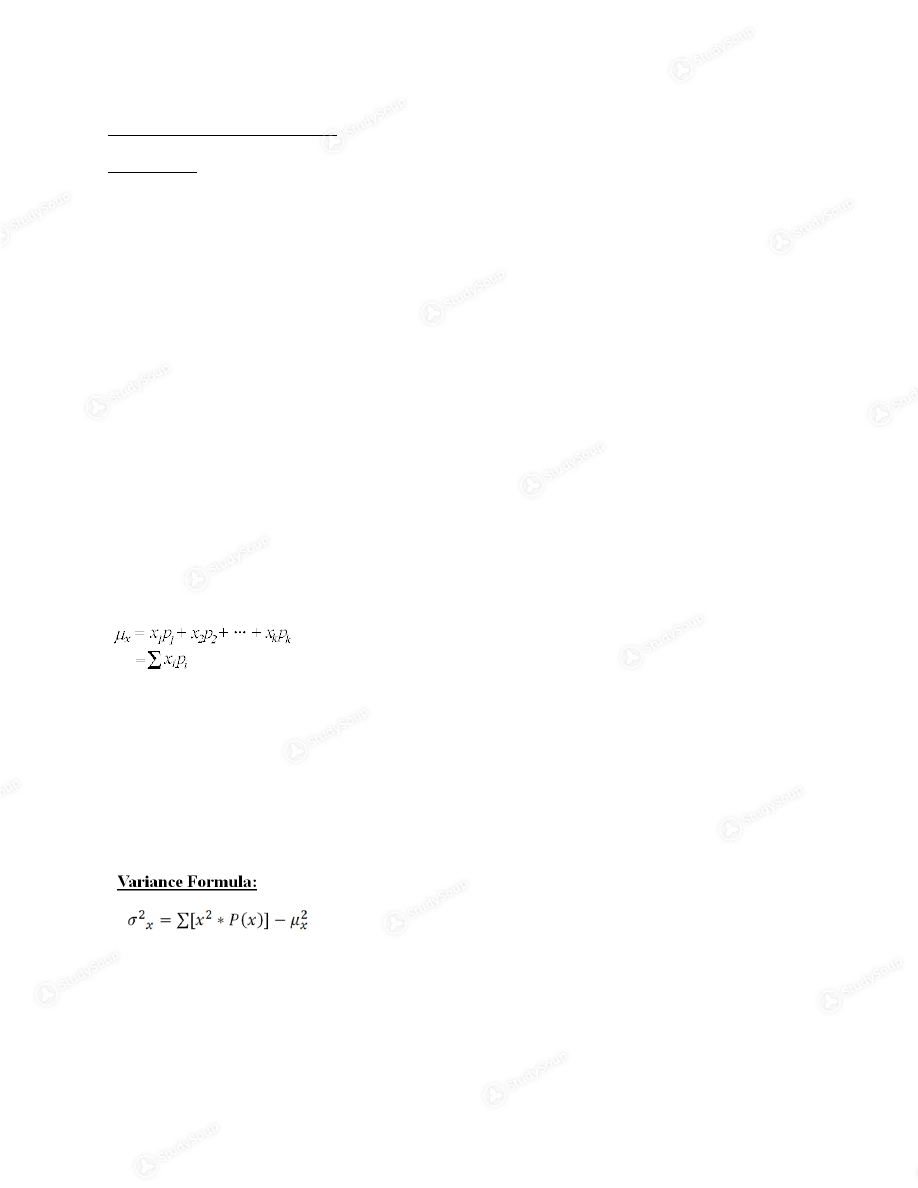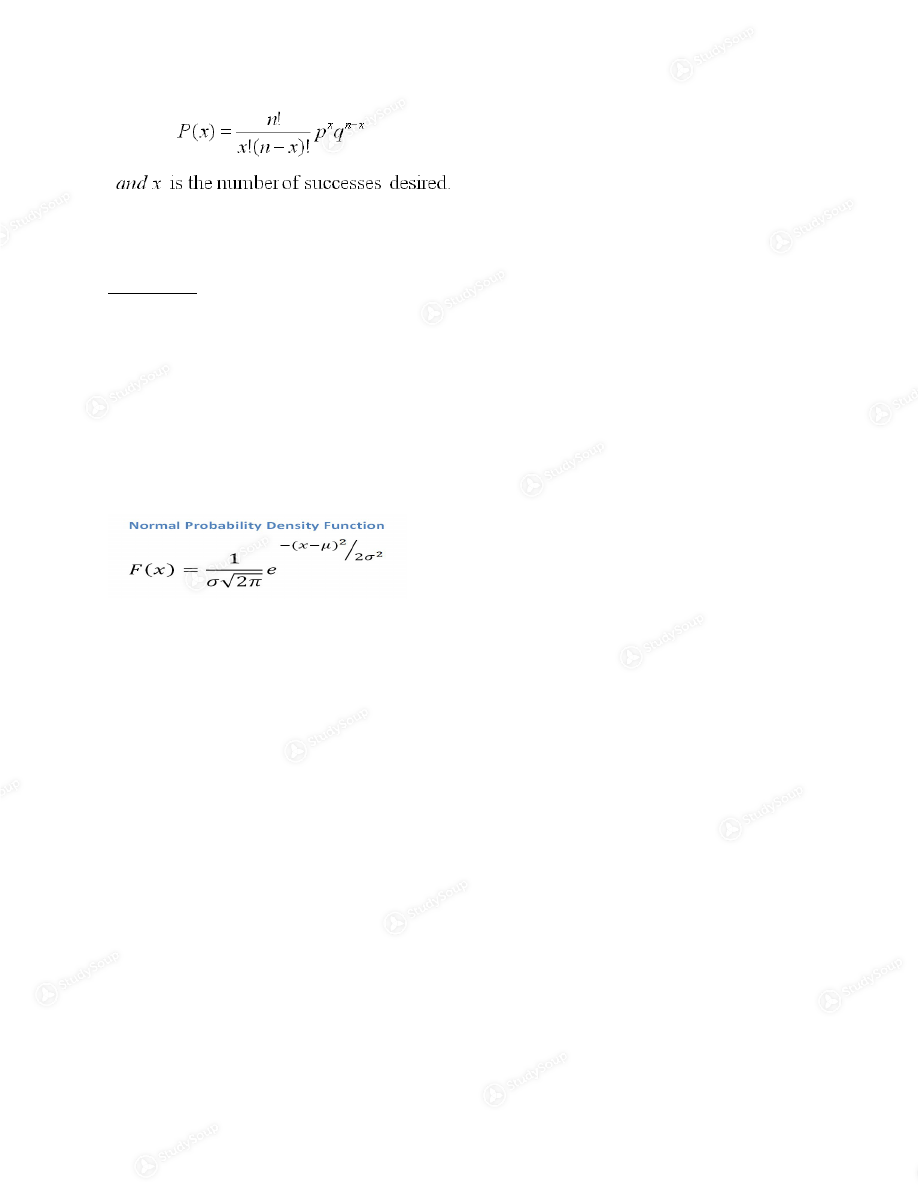Limited time offer 20% OFF StudySoup Subscription details

# OK State - STAT 2013 - Exam 2 Review - Study Guide

### Created by: Morgan Kiser Elite Notetaker

> > > > OK State - STAT 2013 - Exam 2 Review - Study Guide

OK State - STAT 2013 - Exam 2 Review - Study Guide

0 5 3 80 Reviews
This preview shows pages 1 - 2 of a 5 page document. to view the rest of the contentExam 2 Review Chapter 5 Random variable- variable assumes numerical values that are determined by
the outcome of an experiment, where one and only one numerical value is
assigned to each outcome.
Each outcome is determined by chance Discrete random variable-random variable that can only assume a finite or
countable number of values. Values are determined theoretically or by
observation
Continuous random variable- random variable that can assume uncountable
number of values
Discrete includes shoe size, hairs on your head Continuous includes age and temperature Discrete probability distribution- table, graph or formula that gives the
probability associated with each possible value that the random variable can
assume for discrete variable
Mean of discrete random variable Binomial experiment must: Finite number of trials is fixed Only 2 possible outcomes for each trial Probability of success on each trial must remain constant All trials have to be independent of one another Variance of discrete random variable The standard deviation is the positive square root of the varianceChapter 6 Continuous probity distribution is the probability that x will be in a specified
interval of number is the area under the curve corresponding to the interval.
AKA probability curve or probability density function
Must have f(x) greater than or equal to 0 for all values of x and the total area
under the curve must be equal to one
Bell curve is also known as Gaussian distribution or normal distribution Normal Distribution Properties Infinitely large number of normal distributions- mean and standard
deviation
Highest point on normal curve=mean. Also equal to median and mode Symmetrical distribution- curve shape to left go mean is equal to the
shape to the right of the mean
Area to left of mean is equal to mean on right Area is .5 for each Tails extend to infinity and never touch the horizontal axis Z values are found in the chart- measure values to the right To find values to the left, do 1-z Z transformation –transform any normal distribution into standard
distribution

This is the end of the preview. Please to view the rest of the contentJoin more than 18,000+ college students at Oklahoma State University who use StudySoup to get ahead
##### Description: Covers what is on the exam
5 Pages 78 Views 62 Unlocks
• Notes, Study Guides, Flashcards + More!
Get Full Access to OK State - Stat 2013 - Study Guide - Midterm
×
Get Full Access to OK State - Stat 2013 - Study Guide - Midterm

I don't want to reset my password

Need help? Contact support

Need an Account? Is not associated with an account
We're here to help